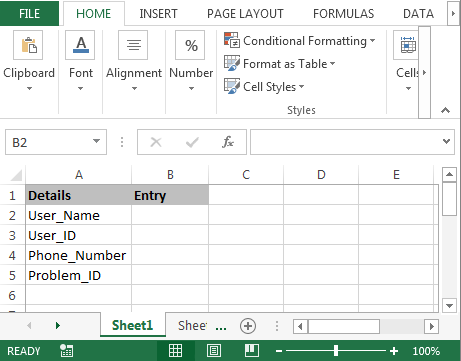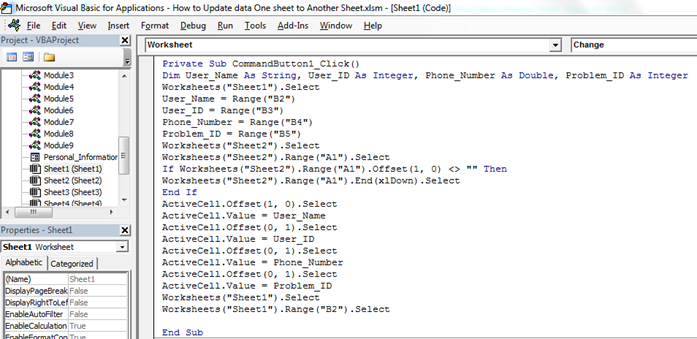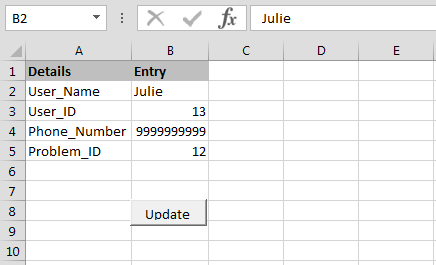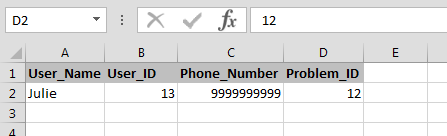How to update data one sheet to Another Sheet through VBA in Microsoft Excel

In this article we are going to learn how to update the data from one sheet to another sheet through VBA in Microsoft Excel.

Let’s take an example to understand how and where we can use this Macro in our data to reduce our daily work.

We want to write this code for those employees who are working in a call center. When clients call them they takes basic details like User_Name, User_ID, Phone_Number, and Problem_ID.

Now we want to write the macro for when a call comes they will note down all the above mentioned details into an excel sheet, and when they click on update that data should be update in another sheet.To update the data from one sheet to another sheet follow below given steps:-
• First we will insert command button in the worksheet.
• Go to Developer tab and then insert Command button from Activexcontrol.
• Assign below mentioned macro:-

```Private Sub CommandButton1_Click()
Dim User_Name As String, User_ID As Integer, Phone_Number As Double, Problem_ID As Integer
Worksheets("Sheet1").Select
User_Name = Range("B2")
User_ID = Range("B3")
Phone_Number = Range("B4")
Problem_ID = Range("B5")
Worksheets("Sheet2").Select
Worksheets("Sheet2").Range("A1").Select
If Worksheets("Sheet2").Range("A1").Offset(1, 0) <> "" Then
Worksheets("Sheet2").Range("A1").End(xlDown).Select
End If
ActiveCell.Offset(1, 0).Select
ActiveCell.Value = User_Name
ActiveCell.Offset(0, 1).Select
ActiveCell.Value = User_ID
ActiveCell.Offset(0, 1).Select
ActiveCell.Value = Phone_Number
ActiveCell.Offset(0, 1).Select
ActiveCell.Value = Problem_ID
Worksheets("Sheet1").Select
Worksheets("Sheet1").Range("B2").Select
End Sub

```

Code Explanation:- First we had defined all the variables. Then we had defined the range for every variables, and then described the range for all the variables. And we had defined that where and in which range data will get update after clicking on Update button.• To run the code press key F5 on the keyboard.
• When you will enter the data and will click on update button then data will get update in another sheet and we can enter new data in the sheet.This is the way we can update the data from one sheet to another sheet through VBA in Microsoft excel.